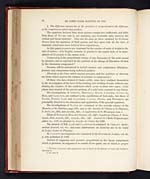# James Clerk Maxwell (1831-1879)

## On the equilibrium of elastic solids

88

MR JAMES CLERK MAXWELL ON THE

2. The difference between two of the pressures is proportional to the difference
of the compressions which they produce.

The equations deduced from these axioms contain two coefficients, and differ
from those of NAVIER only in not assuming any invariable ratio between the
cubical and linear elasticity. They are the same as those obtained by Professor
STOKES from his equations of fluid motion, and they agree with all the laws of
elasticity which have been deduced from experiments.

In this paper pressures are expressed by the number of units of weight to the
unit, of surface; if in English measure, in pounds to the square inch, or in atmo-
spheres of 15 pounds to the square inch.

Compression is the proportional change of any dimension of the solid caused
by pressure, and is expressed by the quotient of the change of dimension divided
by the dimension compressed.*

Pressure will be understood to include tension, and compression dilatation;
pressure and compression being reckoned positive.

Elasticity is the force which opposes pressure, and the equations of elasticity
are those which express the relation of pressure to compression.†

Of those who have treated of elastic solids, some have confined themselves
to the investigation of the laws of the bending and twisting of rods, without con-
sidering the relation of the coefficients which occur in these two cases; while
others have treated of the general problem of a solid body exposed to any forces.

The investigations of LEIBNITZ, BERNOULLI, EULER, VARIGNON, YOUNG, LA
HIRE, and LAGRANGE, are confined to the equilibrium of bent rods; but those of
NAVIER, POISSON, LAMÉ and CLAPEYRON, CAUCHY, STOKES, and WERTHEIM, are
principally directed to the formation and application of the general equations.

The investigations of NAVIER are contained in the seventh volume of the
Memoirs of the Institute, page 373; and in the Annales de Chimie et de Physique,
2e Série,
x.v., 264, and xxxviii., 435; L'Application de la Mécanique, tom. i.

Those of POISSON in Mém. de l' Institut, viii., 429; Annales de Chimie, 2e Série,
xxxvi., 334; xxxvii., 337; xxxviii., 338; xlii. Journal de l'École Polytechnique,
cahier xx., with an abstract in Annates de Chimie for 1829.

The memoir of MM. .LAMÉ and CLAPEYRON is contained in CRELLE'S Mathe-
matical Journal
, vol. vii.; and some observations on elasticity are to be found
in LAMÉ'S Cours de Physique.

M. CAUCHY'S investigations are contained in his Exercises de Analyse, vol. iii.,
p. 180, published in 1828.

Instead of supposing each pressure proportional to the linear compression
which it produces, he supposes it to consist of two parts, one of which is propor–

* The laws of pressure and compression may be found in the Memoir of Lamé and Clapeyron.
See note A.

† See note B.View larger image